# 基于静态场的小学生群体疏散策略仿真研究The Simulation of Evacuation Strategies for Elementary School Students’Group Based on Static Field

• 全文下载: PDF(1301KB)    PP.164-172   DOI: 10.12677/MOS.2018.73020
• 下载量: 416  浏览量: 949   国家自然科学基金支持

As for a lecture hall with single exit, a cellular automata model for the elementary school students’ group with consideration of different evacuation strategies and static field is set up. The numerical simulation is performed to study the relationship between the number of remaining students, the percentage of moving students, and the outflow at the exit with time step. The results show that to maximize the use of the aisle near the chairs and to avoid the jamming when pedestrians select the shortest route will have a significant influence on improving evacuation efficiency.

1. 引言

2. 模型

$\begin{array}{l}A+B+C+D+E+F=1\\ {L}_{p}^{t}=A{l}_{p}^{t}+B{w}_{p}^{t}+C{d}_{p}^{t}+D{a}_{p}^{t}+E{S}_{p}^{t}+F{g}_{p}^{t}\text{\hspace{0.17em}}\text{\hspace{0.17em}}\text{\hspace{0.17em}}\left(p=1,2,\cdots ,9\right)\\ {L}_{i}^{t}=\mathrm{max}\left({L}_{p}^{t}\right)\end{array}$ (1)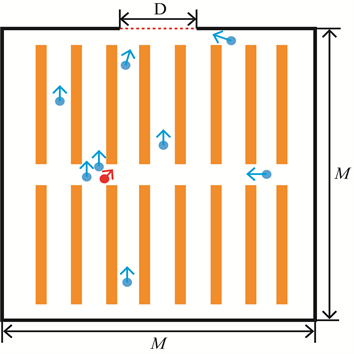Figure 1. Schematic diagram of a lecture hall with single exit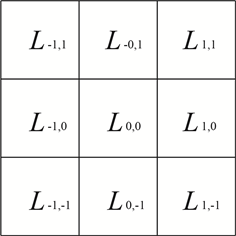(a) (b)

Figure 2. (a) The Moore field; (b) The movement income matrix

1) 距离收益

2) 墙壁收益

3) 桌椅收益

4) 行人相互作用收益

5) 静态场收益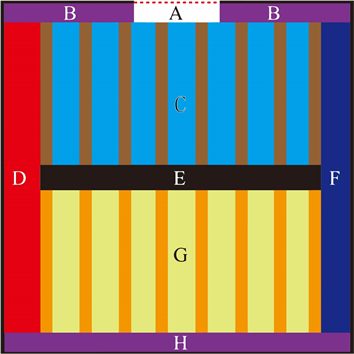Figure 3. The region diagram of static field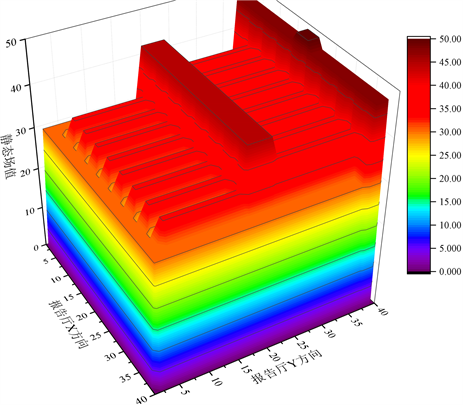(a) (b)

Figure 4. The schematic diagram of static field: (a) static field 1; (b) static field 2

6) 疏散策略收益

3. 模拟结果与讨论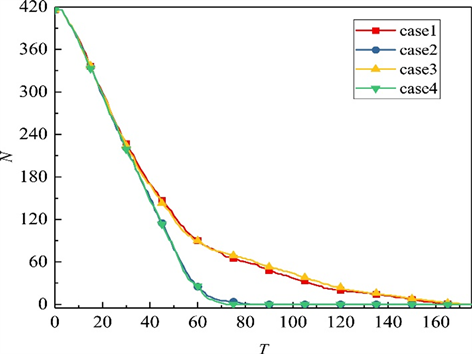Figure 5. The relationship between the number of remaining pedestrians N and the time step T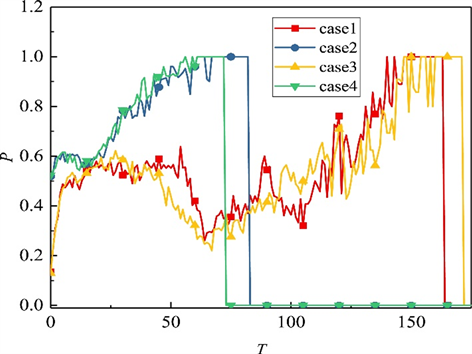Figure 6. The relationship between the moving pedestrian ratio P and the time step T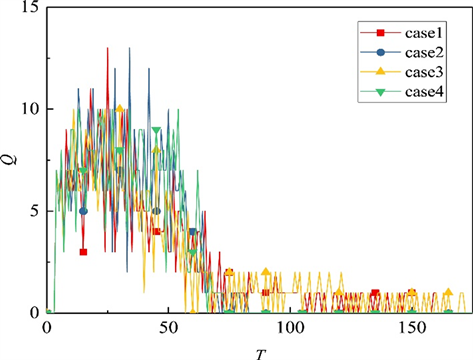Figure 7. The relationship between the exit flow Q and the time step T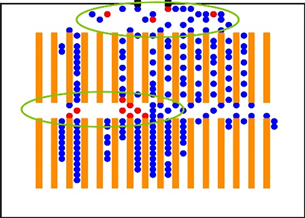(a)(b)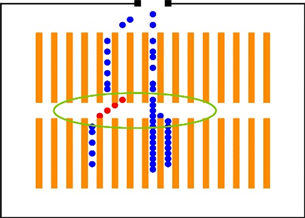(c)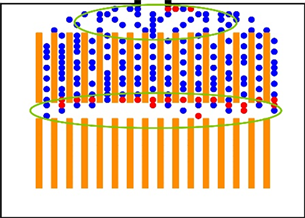(d)(e)(f)

Figure 8. The typical spatiotemporal patterns; case1: (a) t = 30, (b) t = 60, (c) t = 90; case2: (d) t = 30, (e) t = 60, (f) t = 90

4. 结论

  Helbing, D. (2000) Traffic and Related Self-Driven Many-Particle Systems. Physics, 73, 1067-1141.  Henderson, L.F. (1971) The Statistics of Crowd Fluids. Nature, 229, 381-383. https://doi.org/10.1038/229381a0  Hoogendoorn, S.P. (2015) Continuum Theory for Pedestrian Traffic Flow: Local Mute Choice Modelling and Its Implications. Transpoitaion Research Pact C, 59, 183-197. https://doi.org/10.1016/j.trc.2015.05.003  Helbing, D. and Molnár, P. (1995) Social Force Model for Pedestrian Dynamics. Physical Review E Statistical Physics Plasmas Fluids & Related Interdisciplinary Topics, 51, 4282. https://doi.org/10.1103/PhysRevE.51.4282  Helbing, D., Farkas, I. and Vicsek, T. (2000) Simulating Dynamical Features of Escape Panic. Nature, 407, 487-490. https://doi.org/10.1038/35035023  Chraibi, M., Seyfried, A. and Schadschneider, A. (2010) Generalized Centrifugal-Force Model for Pedestrian Dynamics. Physical Review E Statistical Nonlinear & Soft Matter Physics, 82, Article ID: 046111. https://doi.org/10.1103/PhysRevE.82.046111  Burstedde, C., Klauck, K., Schadschneider, A., et al. (2001) Simulation of Pedestrian Dynamics Using a Two-Dimensional Cellular Automaton. Physica A Statistical Mechanics & Its Applications, 295, 507-525. https://doi.org/10.1016/S0378-4371(01)00141-8  Kirchner, A. and Schadschneider, A. (2002) Simulation of Evacuation Processes Using a Bionics-Inspired Cellular Automaton Model for pedestrian Dynamics. Physica A Statistical Mechanics & Its Ap-plications, 312, 260-276. https://doi.org/10.1016/S0378-4371(02)00857-9  Zhang, P., Jian, X.X., Wong, S.C., et al. (2012) Potential Field Cellular Automata Model for Pedestrian Flow. Physical Review E Statistical Nonlinear & Soft Matter Physics, 85, Article ID: 021119. https://doi.org/10.1103/PhysRevE.85.021119  Jian, X.X., Wong, S.C., Zhang, P., et al. (2014) Perceived Cost Potential Field Cellular Automata Model with an Aggregated Force Field for Pedestrian Dynamics. Transportation Research Part C Emerging Technologies, 42, 200-210. https://doi.org/10.1016/j.trc.2014.01.018  Tajima, Y. and Nagatani, T. (2001) Scaling Behavior of Crowd Flow Outside a Hall. Physica A Statistical Mechanics & Its Applications, 292, 545-554. https://doi.org/10.1016/S0378-4371(00)00630-0  Helbing, D., Isobe, M., Nagatani, T., et al. (2003) Lattice Gas Simulation of Experimentally Studied Evacuation Dynamics. Physical Review E, Statistical, Nonlinear, and Soft Matter Physics, 67, Article ID: 067101. https://doi.org/10.1103/PhysRevE.67.067101  Kuang, H., Li, X., Song, T., et al. (2008) Analysis of Pedestrian Dynamics in Counter Flow via an Extended Lattice Gas Model. Physical Review E Statistical Nonlinear & Soft Matter Physics, 78, Article ID: 066117. https://doi.org/10.1103/PhysRevE.78.066117  周金旺, 邝华, 刘慕仁, 孔令江. 成对行为对行人疏散动力学的影响研究[J]. 物理学报, 2009, 58(5): 3001-3007.  田西柱. 基于从众心理的元胞自动机模型[J]. 物联网技术, 2014, 4(4): 83+87.  岳昊. 基于元胞自动机的行人流仿真模型研究[D]: [博士学位论文]. 北京: 北京交通大学, 2009.  尹雪清. 考虑出口区域属性的行人疏散模拟研究[D]: [硕士学位论文]. 呼和浩特: 内蒙古大学, 2014.  李圣男. 基于区块划分元胞自动机模型的行人疏散建模与仿真[D]: [硕士学位论文]. 北京: 北京交通大学, 2015.  菅肖霞. 基于数学和物理机理分析的行人流费用势场元胞自动机模型[D]: [博士学位论文]. 上海: 上海大学, 2013.